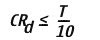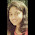THE OP AMP PEAK DETECTOR

Square, triangular, sawtooth, and pulse waves are typical examples of nonsinusoidal waveforms. A conventional ac voltmeter cannot be used to measure these nonsinusoidal waveforms because it is designed to measure the RMS value of the pure sine wave. One possible solution to this problem is to measure the peak values of the nonsinusoidal waveforms.

Figure shows the Circuit Diagram of a PEAK DETECTOR that measures the Positive peak values of the Square wave input. During the positive half-cycle of Vin, the output of the op amp drives D1 on, charging capacitor C to the positive peak value Vp of the input voltage Vin. Thus, when D1 is forward biased, the OP AMP operates as a voltage follower. On the other hand, during the negative half-cycle of Vin, diode D1 is reverse biased, and voltage across C is retained. The only discharge path for C is through Rl since the input bias current Ib is negligible. For proper operation of the circuit, the charging time constant (CRd) and discharging time constant (CRl) must satisfy the following conditions:where,
Rd= Resistance of the Forward biased diode, typically 100Ω
T=time period of the input waveform.

Andwhere Rl is the load resistor.Figure shows the input and output waveform of Peak Detector using OP AMP 741Tittu

Author & Editor

I'm Tittu Thomas, An Electronics and Communication Engineer from India, Kerala. I love doing hobby electronics circuits, blogging, programming, etc. Started this blog while doing my B-Tech degree under CUSAT university.

1 comments so far:

1.What are the applications of a peak detector?

Like Us